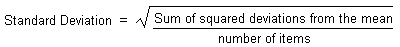HOME MATH DICTIONARY DOWNLOAD FEEDBACK DISCLAIMER
 Question: What is Standard Deviation ? Answer: The standard deviation of a set of numbers, such as heights of people is a measure of how spread out the numbers are from the mean. A natural way to measure spread is as follows: Find how each number deviates from the mean; Since the signs of the deviations, positive or negative, are not of importance (for example 2 above the mean is the same deviation as 2 below the mean) it is sensible to make the deviations positive by squaring them Find the total of the squared deviations Average out this total by dividing it by the number of items (in order to make fair comparison with other sets of data) Take the square root to return to the original units (centimeters, rather than square centimeters in the case of heights) The formula of standard deviation :